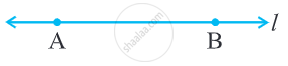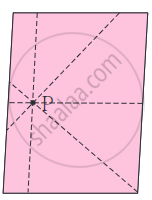Concept of Line

definition

Line: A line is a geometric figure that consists of an infinite number of points lined up straight that extend in both directions forever.

Collinear points: If three or more points lie on the same line, they are called collinear points.

Non-collinear points: If three or more points don't lie on the same line, they are called Non-collinear points.

Line:

• A line is a geometric figure that consists of an infinite number of points lined up straight that extend in both directions forever.

• A line has no endpoints on either side.

• A line is one-dimensional. That is, a line has length, but no width or height.

• A line is uniquely determined by two points.• The line passing through the points A and B is denoted by `
$\overleftrightarrow{AB}$.
• Sometimes small letters l, m, n, etc. will be used to denote lines.

• One and only one line can be drawn through any two distinct points.

• If three or more points lie on the same line, they are called collinear points.

Points not on the same line are the non-collinear points.• An infinite number of lines can be drawn through one point.

• When two or more lines pass through the same point, they are called concurrent lines, and the common point through which they pass is called their point of concurrence.

If you would like to contribute notes or other learning material, please submit them using the button below.

Shaalaa.com

Concept of Line [00:07:24]
S
0%